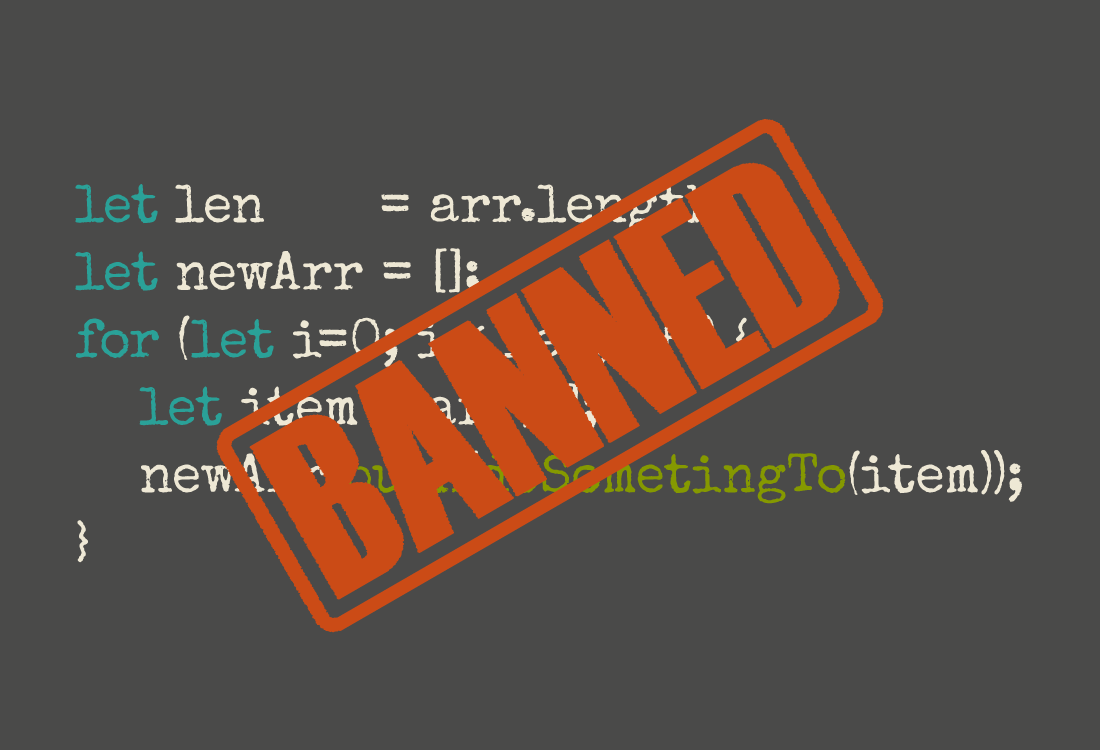## 循环

``````// oodlify :: String -> String
function oodlify(s) {
return s.replace(/[aeiou]/g, 'oodle');
}

const input = [
'John',
'Paul',
'George',
'Ringo',
];
``````

``````let i = 0;
const len = input.length;
let output = [];
while (i < len) {
let item = input[i];
let newItem = oodlify(item);
output.push(newItem);
i = i + 1;
}
``````

``````const len = input.length;
let output = [];
for (let i = 0; i < len; i = i + 1) {
let item = input[i];
let newItem = oodlify(item);
output.push(newItem);
}
``````

``````let output = [];
for (let item of input) {
let newItem = oodlify(item);
output.push(newItem);
}
``````

## mapping

for...of 循环比 for 循环更清晰，但是依然需要一些配置性的代码。如不得不初始化一个 output 数组并且每次循环都要调用 push() 函数。但有办法可以让代码更加简洁有力，我们先扩展一下问题。

``````const fellowship = [
'frodo',
'sam',
'gandalf',
'aragorn',
'boromir',
'legolas',
'gimli',
];

const band = [
'John',
'Paul',
'George',
'Ringo',
];
``````

``````let bandoodle = [];
for (let item of band) {
let newItem = oodlify(item);
bandoodle.push(newItem);
}

let floodleship = [];
for (let item of fellowship) {
let newItem = oodlify(item);
floodleship.push(newItem);
}
``````

``````function oodlifyArray(input) {
let output = [];
for (let item of input) {
let newItem = oodlify(item);
output.push(newItem);
}
return output;
}

let bandoodle = oodlifyArray(band);
let floodleship = oodlifyArray(fellowship);
``````

``````function izzlify(s) {
return s.replace(/[aeiou]+/g, 'izzle');
}
``````

``````function oodlifyArray(input) {
let output = [];
for (let item of input) {
let newItem = oodlify(item);
output.push(newItem);
}
return output;
}

function izzlifyArray(input) {
let output = [];
for (let item of input) {
let newItem = izzlify(item);
output.push(newItem);
}
return output;
}
``````

``````function map(f, a) {
let output = [];
for (let item of a) {
output.push(f(item));
}
return output;
}
``````

``````function map(f, a) {
if (a.length === 0) { return []; }
return [f(a)].concat(map(f, a.slice(1)));
}
``````

``````let bandoodle     = band.map(oodlify);
let floodleship   = fellowship.map(oodlify);
let bandizzle     = band.map(izzlify);
let fellowshizzle = fellowship.map(izzlify);
``````

## reducing

``````const heroes = [
{name: 'Hulk', strength: 90000},
{name: 'Spider-Man', strength: 25000},
{name: 'Hawk Eye', strength: 136},
{name: 'Thor', strength: 100000},
{name: 'Black Widow', strength: 136},
{name: 'Vision', strength: 5000},
{name: 'Scarlet Witch', strength: 60},
{name: 'Mystique', strength: 120},
{name: 'Namora', strength: 75000},
];
``````

``````let strongest = {strength: 0};
for (hero of heroes) {
if (hero.strength > strongest.strength) {
strongest = hero;
}
}
``````

``````let combinedStrength = 0;
for (hero of heroes) {
combinedStrength += hero.strength;
}
``````

``````function greaterStrength(champion, contender) {
return (contender.strength > champion.strength) ? contender : champion;
}

function addStrength(tally, hero) {
return tally + hero.strength;
}

const initialStrongest = {strength: 0};
let working = initialStrongest;
for (hero of heroes) {
working = greaterStrength(working, hero);
}
const strongest = working;

const initialCombinedStrength = 0;
working = initialCombinedStrength;
for (hero of heroes) {
working = addStrength(working, hero);
}
const combinedStrength = working;
``````

``````function reduce(f, initialVal, a) {
let working = initialVal;
for (item of a) {
working = f(working, item);
}
return working;
}
``````

reduce 模式在 JavaScript 中也是很常用的，因此 JavaScript 为数组提供了内置的方法，不需要自己来写。通过内置方法，代码就变成了：

``````const strongestHero = heroes.reduce(greaterStrength, {strength: 0});
const combinedStrength = heroes.reduce(addStrength, 0);
``````

ok，如果足够细心的话，你会注意到上面的代码其实并没有短很多。不过也确实比自己手写的 reduce 代码少写了几行。但是我们的目标并不是使代码变短或者少写，而是降低代码复杂度。现在的复杂度降低了吗？我会说是的。把处理每个元素的代码和处理循环代码分离开来了，这样代码就不会互相纠缠在一起了，降低了复杂度。

reduce 方法乍一看可能觉得非常基础。我们举的 reduce 大部分也比如做加法这样的简单例子。但是没有人说 reduce 方法只能返回基本类型，它可以是一个 object 类型，甚至可以是另一个数组。当我第一次意识到这个问题的时候，自己也是豁然开朗。所以其实可以用 reduce 方法来实现 map 或者 filter，这个留给读者自己做练习。

## filtering

``````const heroes = [
{name: 'Hulk', strength: 90000, sex: 'm'},
{name: 'Spider-Man', strength: 25000, sex: 'm'},
{name: 'Hawk Eye', strength: 136, sex: 'm'},
{name: 'Thor', strength: 100000, sex: 'm'},
{name: 'Black Widow', strength: 136, sex: 'f'},
{name: 'Vision', strength: 5000, sex: 'm'},
{name: 'Scarlet Witch', strength: 60, sex: 'f'},
{name: 'Mystique', strength: 120, sex: 'f'},
{name: 'Namora', strength: 75000, sex: 'f'},
];
``````

ok，现在有两个问题，我们想要：

1. 找到所有的女性英雄；
2. 找到所有能量值大于500的英雄。

``````let femaleHeroes = [];
for (let hero of heroes) {
if (hero.sex === 'f') {
femaleHeroes.push(hero);
}
}

let superhumans = [];
for (let hero of heroes) {
if (hero.strength >= 500) {
superhumans.push(hero);
}
}
``````

``````function isFemaleHero(hero) {
return (hero.sex === 'f');
}

function isSuperhuman(hero) {
return (hero.strength >= 500);
}

let femaleHeroes = [];
for (let hero of heroes) {
if (isFemaleHero(hero)) {
femaleHeroes.push(hero);
}
}

let superhumans = [];
for (let hero of heroes) {
if (isSuperhuman(hero)) {
superhumans.push(hero);
}
}
``````

``````function filter(predicate, arr) {
let working = [];
for (let item of arr) {
if (predicate(item)) {
working = working.concat(item);
}
}
}

const femaleHeroes = filter(isFemaleHero, heroes);
const superhumans  = filter(isSuperhuman, heroes);
``````

``````const femaleHeroes = heroes.filter(isFemaleHero);
const superhumans  = heroes.filter(isSuperhuman);
``````

## finding

filter 已经信手拈来了吧。这时如果只想找一个英雄该怎么办？比如找 “Black Widow”。使用 filter 会这样写：

``````function isBlackWidow(hero) {
return (hero.name === 'Black Widow');
}

const blackWidow = heroes.filter(isBlackWidow);
``````

``````function find(predicate, arr) {
for (let item of arr) {
if (predicate(item)) {
return item;
}
}
}

const blackWidow = find(isBlackWidow, heroes);
``````

``````const blackWidow = heroes.find(isBlackWidow);
``````

find 再次体现了四两拨千斤的特点。通过 find 方法，把问题简化为：你只要关注如何判断你要找的东西就可以了，不必关心迭代到底怎么实现等细节问题。

## 总结

1. 消除了循环结构，使得代码变的简洁易读；
2. 通过适当的方法名称来描述我们使用的模式，也就是：map，reduce，filter 和 find；
3. 把问题从处理整个数组简化到处理每个元素。